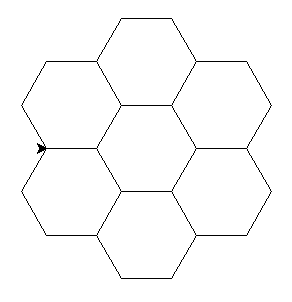# 🐍 🐢

In this lesson, we will be drawing with the turtle module.

Run Python in interactive mode (write Python in the command line).

```\$ python

>>>
```

The characters `>` and `\$` are printed by the computer, not by you. On Windows, it will be `>` instead of `\$`. Before the `\$` or `>`, there can be some other words.

Then write:

```from turtle import forward

forward(50)
```

Now a popup window will appear, don't close it. Place it somewhere where you will be able to see it and your command line, too.

If you are a Mac OS X user and have problems with errors like: `ModuleNotFoundError: No module named '_tkinter'` then TKInter was not yet installed on your system You will need to install it manually by adding following command in your terminal: brew install python-tk@3.(your python version) you can check your version by command python3 --version (it should be for example 3.11.3) so the first command should be for example: brew install python-tk@3.11.3

## Where is the turtle?

Currently, the turtle is disguised as an arrow. There is a way how to unmask it:

```from turtle import shape

shape('turtle')
```

## Rotation

The turtle can rotate and crawl across the "paper". It has a brush on its tail which draws a line.

```from turtle import left, right

forward(50)
left(60)
forward(50)
right(60)
forward(50)
```

Now give the turtle some commands. If you don't like the drawing you can close the window, or import and use the function `clear()`.

## Turtle program

Interactive mode is good for trying new stuff but we will now go back to our editors and write some program in a file.

Create a file `~/pyladies/03/drawing.py`.

The directory `~/pyladies` can have a different name on your laptop – see Python installation.

You can have a different name for your file, just don't use `turtle.py`.

Write drawing commands into the file and in the end call the function `exitonclick` (imported from module `turtle`).

Question

What does the function `exitonclick` do?

If you are using directly the VS Code terminal for running turtle,

instead of using `exitonclick`, use `turtle.done()` to "start the main program" as the last command of your turtle script.

### Square

Draw a square.A square has 4 equal straight sides and 4 90° angles.

### Rectangle

Draw a rectangle.

Try to make it so that the turtle will "look" to the right in the end (like it was in the beginning).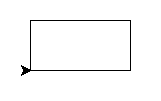### Three squares

Now draw three sqares, each rotated by 20°.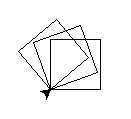### Can we write it better?

So much code! There has to be a way how to simplify it.

There is. Now we will learn the command `for`.

## Repetition

What does the following code do? Save it as `~/pyladies/03/loop.py`

```for number in range(5):
print(number)

for greeting in 'Ahoj', 'Hello', 'Hola', 'Hei', 'SYN':
print(greeting + '!')
```

What does the command `for` do?

### Overwriting variables

What does the following program do?

```sum = 0

for number in 8, 45, 9, 21:
sum = sum + number

print(sum)
```

### Square

Back to drawing! This time we will use loops.

Draw a square.

Use `forward` only twice, once in the import and once as function.### Discontinuous line

The functions `penup` and `pendown` from the `turtle` module tell the turtle to stop/start drawing.

Try to draw a discontinuous line.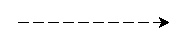### Solution

Now try to make it so that the lines that are drawn become gradually bigger.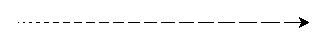Help

What exactly does the command `for` do? Can we use the variable that it sets up?

### Solution

Loop variable Naming

Always use a meaningful loop variable name, like for index_tab_browser in range(3, 18): close_tab_in_browser(index_tab_browser) not just i, j, x, y etc. When using meaningful names:

• it's easier to find errors in the loop logic
• text searches for the variable name return relevant pieces of code operating on the same data are more reliable

There is one exception - when it's a single-level loop and the variable has no meaning other than "the number of times I've been through this loop", in which case `i` can be used.

### Three squares

Finally, draw 3 squares, each rotated by 20°. Now you know how to write it in a simple way: repeat the code by using `for`, do not copy the code.### Solution